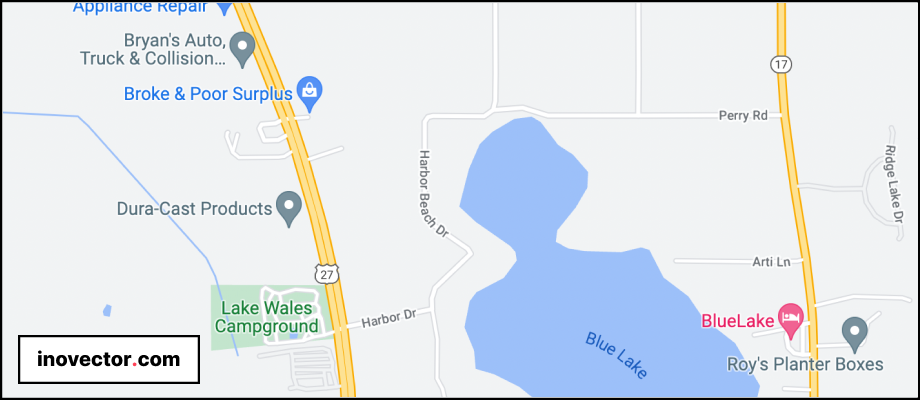# Get locations nearest the user location with MySQL/PHP in LaravelDumitru Botezatu
22 Aug, 2019

Recently, for a project, I had the task of showing all the locations from the database on the map depending on where the user is and, at the same time, the user was able to choose the distance: 1km, 5km, 10km, etc.

We thought about sharing this experience with you. So, let's get to the code.

### Example with Mysql:

Table restaurants:

``SELECT *, ( 6371 * acos( cos( radians(40.6591158) ) * cos( radians( lat ) ) * cos( radians( lng ) - radians(-73.7841042,14) ) + sin( radians(40.6591158) ) * sin( radians( lat ) ) ) ) AS distance FROM restaurants HAVING distance < 1;``

To search by miles instead of kilometers, replace 6371 with 3959.

### Example query with Laravel Eloquent:

``````Restaurants::query()
->whereRaw('6371 * acos( cos( radians(40.6591158) ) * cos( radians( lat ) ) * cos( radians( lng ) - radians(-73.7841042,14) ) + sin( radians(40.6591158) ) * sin( radians( lat ) ) ) )  < 1')
->get();``````

So we will receive a list of restaurants from the database that is within one km from the user's location.

### Example with Collection in Laravel:

1. We will calculate the distance between the user and each restaurant using the Haversine formula.

2. We will create a new collection with new parameter "distance"

3. Then we will condition this array to obtain only restaurants with a distance of less than 1km.

``````function calculateDistanceBetweenTwoAddresses(\$lat1, \$lng1, \$lat2, \$lng2){

\$delta_lat = \$lat2 - \$lat1;
\$delta_lng = \$lng2 - \$lng1;

\$hav_lat = (sin(\$delta_lat / 2))**2;
\$hav_lng = (sin(\$delta_lng / 2))**2;

\$distance = 2 * asin(sqrt(\$hav_lat + cos(\$lat1) * cos(\$lat2) * \$hav_lng));

\$distance = 6371*\$distance;
// If you want calculate the distance in miles instead of kilometers, replace 6371 with 3959.

return \$distance;
}``````

Create new collection of markers:

``````\$markers = collect(\$restaurants)->map(function(\$item) {
\$item['distance'] = calculateDistanceBetweenTwoAddresses(\$restaurant['lat'], \$restaurant['lng'], \$userLat, \$userLng);

return \$item;
});``````

After we have created a new collection in which each restaurant has the value of the distance between the user and the restaurant, we can now condition this collection to obtain only the restaurants within the radius chosen by the user, in our case 1 Km.

``\$restaurants = \$markers->where('distance', '<', 1);``
CONTENTS

25 Jan, 2023

22 Aug, 2022

19 Jul, 2022

22 Jun, 2022

29 Apr, 2022

22 Feb, 2022

04 Feb, 2022

21 Jan, 2022

06 Jan, 2022

20 Dec, 2021

24 Nov, 2021

01 Feb, 2021